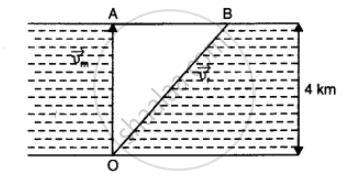CBSE (Science) Class 11CBSE
Share

# A Man Can Swim with a Speed of 4.0 Km/H in Still Water. How Long Does He Take to Cross a River 1.0 Km Wide If the River Flows Steadily at 3.0 Km/H and He Makes His Strokes Normal to the River Current? How Far Down the River Does He Go When He Reaches the Other Bank - CBSE (Science) Class 11 - Physics

#### Question

A man can swim with a speed of 4.0 km/h in still water. How long does he take to cross a river 1.0 km wide if the river flows steadily at 3.0 km/h and he makes his strokes normal to the river current? How far down the river does he go when he reaches the other bank?

#### Solution 1

Hera, vecV_m = 4 km h^(-1), vecv_r = 3 km h^(-1)

OA = 1 km

Let t = time taken by man to reach the other bank

then t = (OA)/v_m = 1/4 = 0.25 h

Distance AB = v_t xx t =3 xx 0.25 = 0.75 km.#### Solution 2

Speed of the man, vm = 4 km/h

Width of the river = 1 km

Time taken to cross the river = "Width of river"/"Speed of man"

=1/4h = 1/4 xx 60 = 15 min

Speed of the river, vr = 3 km/h

Distance covered with flow of the river = vr × t

= 3 xx 1/4 = 3/4 km

=3/4 xx 1000 = 750 m

Is there an error in this question or solution?

#### APPEARS IN

NCERT Solution for Physics Textbook for Class 11 (2018 to Current)
Chapter 4: Motion in a Plane
Q: 13 | Page no. 86
Solution A Man Can Swim with a Speed of 4.0 Km/H in Still Water. How Long Does He Take to Cross a River 1.0 Km Wide If the River Flows Steadily at 3.0 Km/H and He Makes His Strokes Normal to the River Current? How Far Down the River Does He Go When He Reaches the Other Bank Concept: Projectile Motion.
S